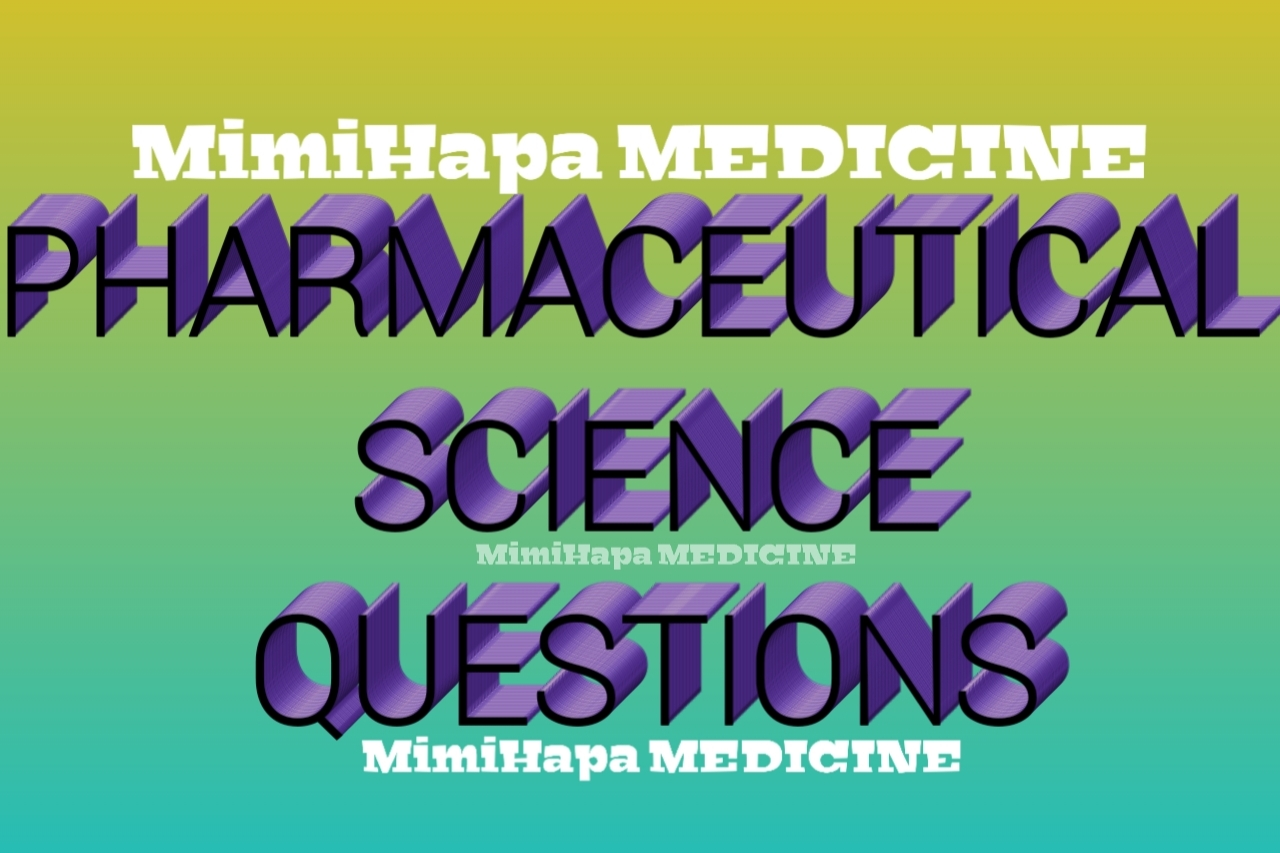# Pharmaceutical Inorganic Chemistry Questions For Pst Nta Level 4 | Pharmacy QuestionsMimiHapa MEDICINE as HOME OF MEDICINE Share with medical student of pharmacy known as pharmaceutical science course (PST) a collection of past paper questions of pharmacy for subject of These Pharmaceutical Inorganic Chemistry Questions For Pst Nta Level 4, These are collections of question from the previously past paper, As Pharmacy Questions Time. So below are those question of Pharmaceutical Inorganic Chemistry can pass through it and try to solve those question and be completely on it (module)

1.  What is buffer solution?

2.  What is buffer capacity?

3.  What are the features of buffer solutions?

4.  What are the uses of buffer solution?

5.  What are the group three elements?

6.  What are the physical characteristics of group three elements?

7.  What are the chemical characteristics of group three elements?

8.  What are the uses of Group three elements in pharmacy?

9.  What are the transition elements?

10.     What are the physical characteristics of Transition elements?

11.     What are the chemical characteristics of transition elements?

12.     What are the uses of transition elements?

13.     What are the group two elements?

14.     What are the physical characteristics of group two elements?

15.     What are the chemical characteristics of group two elements?

16.     What is the importance of group two elements?

17.     What are the group one elements?

18.     What are the physical characteristics of group one elements?

19.     What are the chemical characteristics of group one elements?

20.     What are the importances of group one elements?

21.     What is periodic table?

22.     What is the periodic law?

23.     What are the trends of elements in a periodic table?

24.     What is electronic configuration?

25.     What are quantum numbers?

26.     What are atomic orbitals?

27.     What are the rules used in filling electrons in orbital?

28.     What is an Atom?

29.     What is the Dalton’s Atomic Theory?

30.     What is the Composition of an Atom?

31.     Where do the Protons, Neutrons and Electrons found in an Atom?

32.     What is the Difference between Atomic Number and Mass Number?

33.     What is an isotopes?

34.     What is pharmaceutical chemistry?

35.     What are the branches of pharmaceutical chemistry?

36.     What is pharmaceutical inorganic chemistry?

37.     What is the importance of chemistry in pharmacy?

38.     What are the group four elements?

39.     What are the physical characteristics of group four elements?

40.     What are the chemical characteristics of group four elements?

41.     What is the importance of Group four elements?

42.     What are the group five elements?

43.     What are the physical characteristics of group five elements?

44.     What are the chemical characteristics of group five elements?

45.     What are the uses of Group Five elements?

46.     What are the group six elements?

47.     What are the physical characteristics of group six elements?

48.     What are the chemical characteristics of group six elements?

49.     What are the uses of Group six elements?

50.     What are the group seven elements?

51.     What are the physical characteristics of group seven elements?

52.     What are the chemical characteristics of group seven elements?

53.     What is the importance of Group seven elements?

54.     What are the group eight elements?

55.     What are the physical characteristics of group eight elements?

56.     What are the chemical characteristics of group eight elements?

57.     What are the uses of Group eight elements?

58.     What are the rare earth elements?

59.     What are the physical characteristics of rare earth elements?

60.     What are the chemical characteristics of rare earth elements?

61.     What is the importance of rare earth elements?

62.     What is matter?

63.     How do we classify matter?

64.     What is a compound?

65.     What are complex compounds?

66.     How do we name compounds?

67.     What are the characteristics of compounds?

68.     What is mixture?

69.     What are the characteristics of mixtures?

70.     What are the methods used to separate mixture?

71.     What is the meaning of physical characteristics of drug?

72.     What is the meaning of chemical characteristics of drug?

73.     State the physical and chemical characteristics of drug

74.     What is Acid?

75.     How do we classify acids?

76.     What are the properties of acid?

77.     What is the importance of acid in pharmacy?

78.     What is a base?

79.     What are the properties of a base?

80.     What is the importance of a base in pharmacy?

81.     What is Salt?

82.     How do we categorize salt?

83.     What are the characteristics of a salt?

84.     What are the importance of salt in pharmacy?

85.     What are the differences between mixtures and compounds?

86.     Explain the following methods for separating mixtures:

i.     Distillation

ii.     Fractional distillation

iii.     Magnet